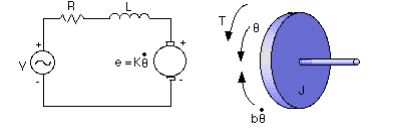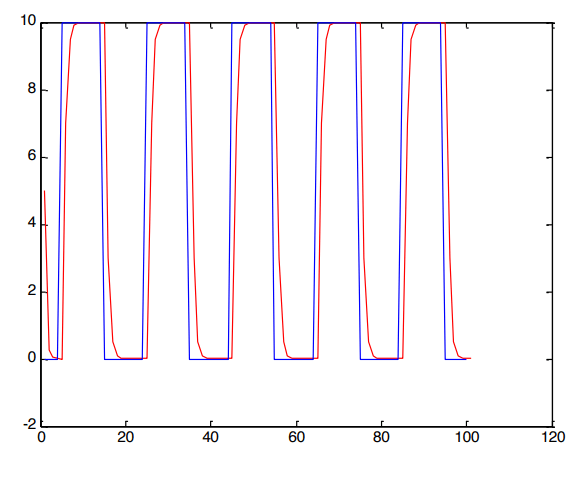# DC Motor Speed Control Solved

35.00 \$ 17.50 \$

Category:
Click Category Button to View Your Next Assignment | Homework

You'll get a: . ` zip` file solution, download link after Payment

## Description

1. Physical Setup and System Equations
A common actuator in control systems is the DC motor. It directly provides rotary motion and, coupled with wheels or drums and cables, can provide transitional motion. The electric circuit of the armature and the free body diagram of the rotor are shown in the following figure:From the figure above we can write the following equations based on Newton’s law combined with Kirchhoff’s law:Where the physical parameters are:* Moment of inertia of the rotor (J) = 0.01 kg.m^2/s^2
* Damping ratio of the mechanical system (b) = 0.1 Nms
* Electromotive force constant (K) = 0.01 Nm/Amp
* Electric resistance (R) = 1 ohm
* Electric inductance (L) = 0.5 H
* Input (V): Source Voltage

In the state-space form, the equations above can be expressed by choosing the rotational speed and electric current as the state variables and the voltage as an input. The output is chosen to be the rotational speed.2. Project Requirements and Descriptions

2.1 Control Objective:
The objective of this project is to design a digital controller that makes the speed of the motor follow a desired speed (reference signal).

2.2 Procedures
1. Define
Describe the continuous system using the state-space form dx/dt=Fx+Gu,
y=Hx+Ju.

Use the following commands to represent the system in Matlab.
J=0.01;
b=0.1;
K=0.01;
R=1;
L=0.5;
F=[-b/J K/J -K/L -R/L];
G=[01/L];
H=[1 0];
J=0;

2. Assume the sample time T=0.5. Obtain the Discrete Equivalent of the continuous system (use ZOH). Determine in the following discrete-time system

3. Use state-space method to design
• a controller gain K (assume desired poles are 0.1 and 0.1)
• an observer (Lp) (assume desired poles are 0.2 and 0.2)
• Nx and Nu
• The controller using the estimated states for a reference signal r(k)

4. Suppose the desired speed r(k)=100 is a constant. Use Matlab to simulate the response of the closed-loop system. Plot the speed of the motor (i.e. x1) and the reference signal.
5. Suppose the reference signal r(k) is a square ware. (i.e. we want the speed of the motor track the square wave) use the following codes to generate the square wave.
for k=1:100;
r(k)=10;
if mod(round(k/10),2)==0
r(k)=0;
end;
end;

Conduct the computer simulation and plot the speed of the motor and reference signal.

6. Assume there is a disturbance d(k) satisfying d(k)=0 when k is less than 15 and d(k)=0.05 when k is greater than and equals to 15 in the system, i.e.,
What will the simulation result of the controller you designed in previous steps?

7. In the presence of the disturbance, design an integral controller for a better result if all x(k) are measurable. What if only the output is available? (Note that d(k) is not available for us to design the estimator.) Design an estimator and use the estimated states in the integral controller for simulation. Does the output converge to the reference signal?

Show detailed design, Matlab scripts, plots, and observations/remarks.
A sample output for problem 5.